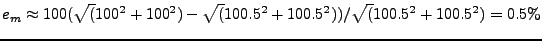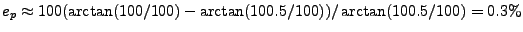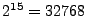Next: Interpolation Kernels Up: Implementation Previous: Implementation   Contents

## Output formats

Computations are done in complex float. Casting these values to complex short format introduces an error. Of course, the main advantage is a factor 2 reduction in the size of the output file.

Now an example follows for the error in amplitude and phase for a complex value of about (100,100). If the actual interpolated complex value equals (100.5,100.5), then the error in the magnitude approximately is(U1)

If the actual value did equal (100.5,100.0), then the error in the phase is approximately(U2)

These are worst case scenarios. If the complex value is larger, then the relative error decreases. Note that the maximum for a signed short integer is.

Leijen 2009-04-14